## Exploring a New Model for Neutrino Propagation Using Current and Future Experiments

Abstract

The existence of neutrinos has been known for nearly a century, yet the exact behavior of these particles is still unclear. Among the many questions surrounding them is the question of how exactly they propagate through space. The traditional propagation model is unitary, having real eigenvalues for the free neutrino Hamiltonian. However, recent discussion in the literature has pointed toward the possibility of a new, more detailed model---namely, one that is non-unitary. We explore the framework of one such model in which neutrinos couple to light decay states, having instead complex Hamiltonian eigenvalues. This gives rise to nine new propagation parameters in three distinct groups. We compare this model both to Tokai--to--Kamioka data and to potential future data from the Jiangmen Underground Neutrino Observatory. Specifically, we obtain constraints on the new non-unitary parameters to test the model's feasibility.

Introduction

Neutrinos oscillate between different flavors as they propagate.

Neutrinos have 3 flavor states and 3 mass states. Each flavor state $$\lvert \nu_\alpha \rangle$$ can be written in terms of the 3 mass states $$\lvert \nu_i \rangle$$,

$$\lvert \nu_\alpha \rangle = \sum_i U_{\alpha i} \lvert \nu_i \rangle$$,

where $$\alpha = e, \mu, \tau$$ and $$i = 1, 2, 3$$. The matrix $$U$$ is unitary and is at the center of the traditional neutrino propagation model.

Current and future experiments that measure neutrino oscillations can be modeled.

$$\bar{P}_{\alpha \beta} = \left( \int_{E_i}^{E_f} dE \frac{d\Phi}{dE} \sigma(E) P_{\alpha \beta}(E) \right) \Big / \left(\int_{E_i}^{E_f} dE \frac{d\Phi}{dE} \sigma(E) \right)$$

The average transition probability $$\bar{P}_{\alpha \beta}$$ from flavor $$\alpha$$ to $$\beta$$ is modeled by calculating the fraction of detected neutrinos with oscillations over that without oscillations.

Two experiments that can model these oscillations are Tokai-to-Kamioka (T2K) and the Jiangmen Underground Neutrino Observatory (JUNO)

The T2K experiment measures the average $$\mu$$-to-$$e$$ neutrino oscillations over the entire energy spectrum of incoming neutrinos. JUNO is expected to measure electron neutrino survival ($$e$$-to-$$e$$) probabilities over a range of energies.

The expected number of detection events (flux times cross section) was estimated using the curves provided by the T2K  and JUNO  collaborations. The baseline distances for neutrino travel are 295 km (T2K) and 53 km (JUNO).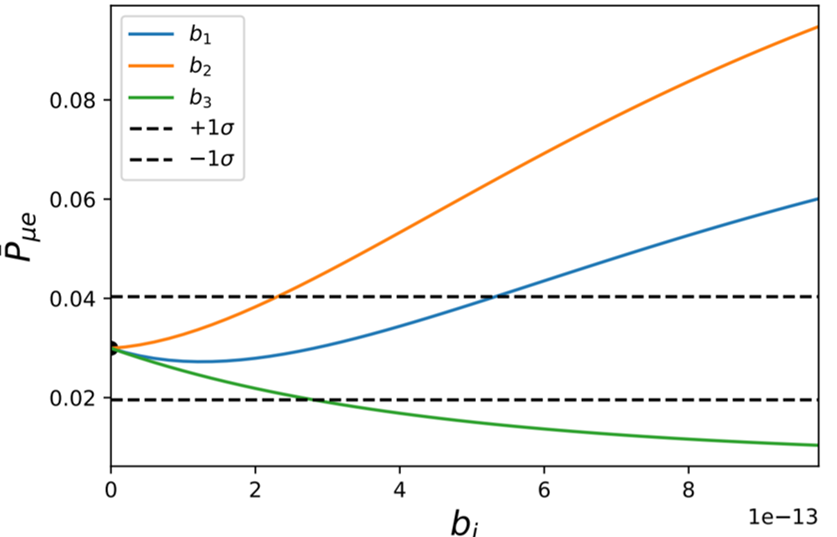Fig 1a: The T2K model is expected to be sensitive to all $$b_i$$. All other parameters were turned off.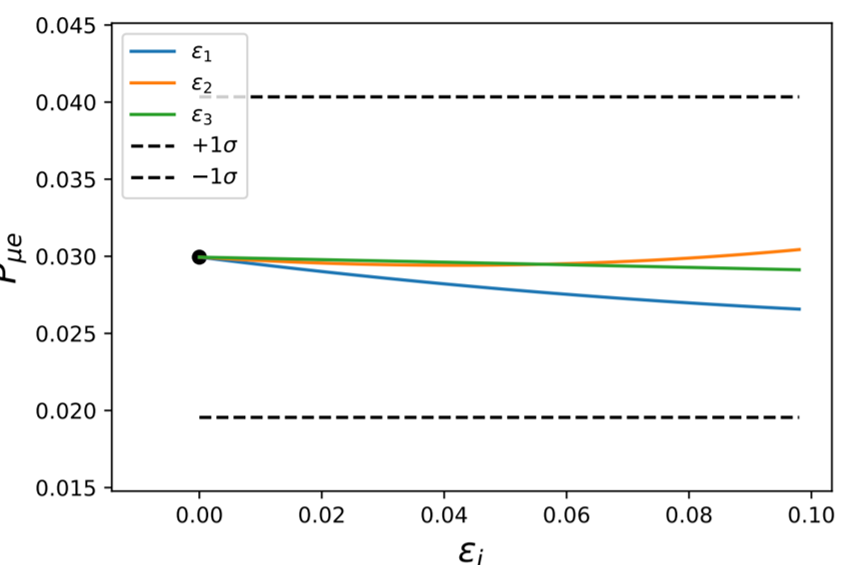Fig 1b: No sensitivity is expected from the $$\epsilon_i$$. The $$b_i = 10^{-13}$$ eV.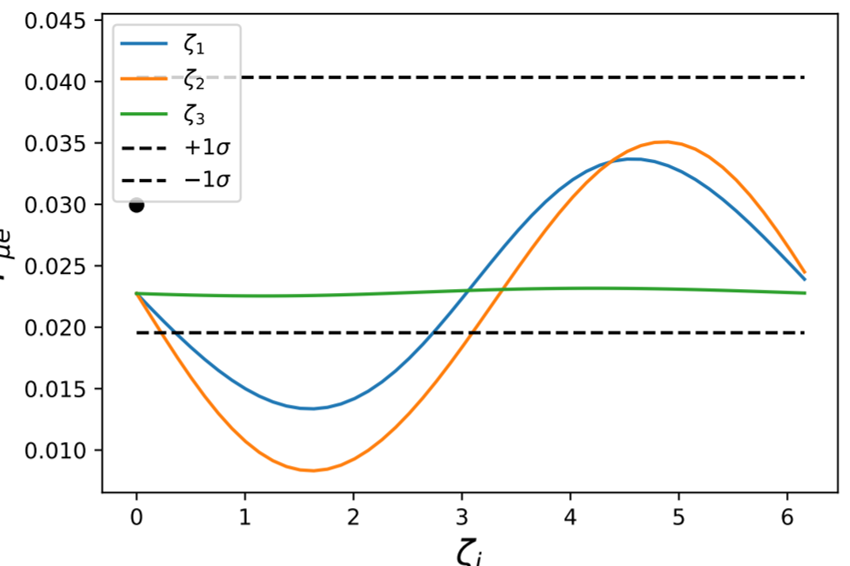Fig 1c: Mild sensitivity is expected from the $$\zeta_i$$. The $$b_i = 10^{-13}$$ eV and the $$\epsilon_i = 0.1$$.

Results from T2K

This non-unitary model can explain current data on neutrinos from the Tokai-to-Kamioka experiment.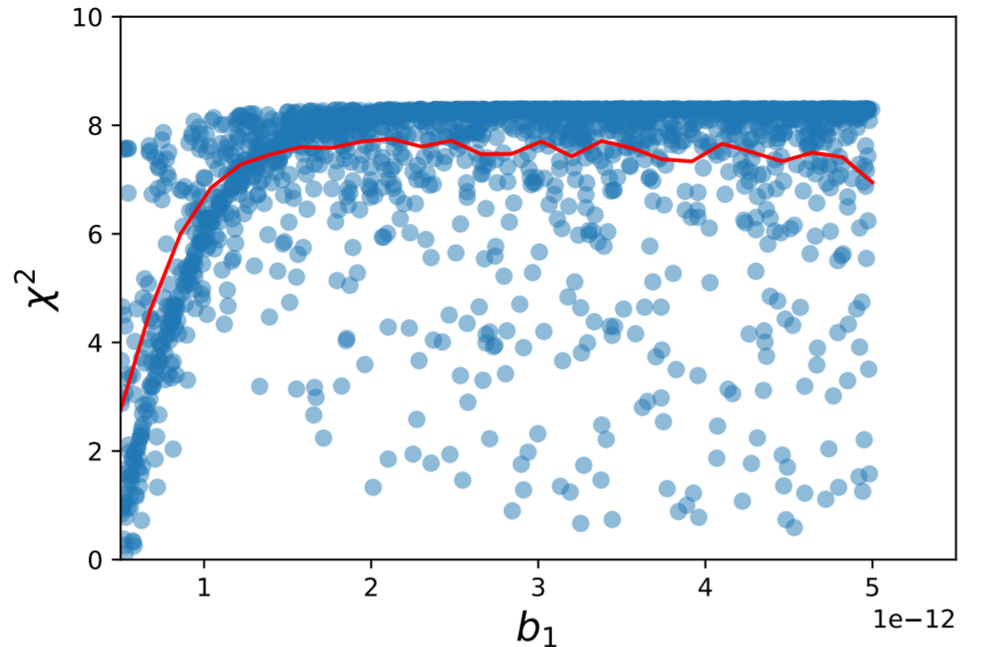Fig 2: $$\chi^2$$ values as a function of one $$b$$ parameter (in eV) while all other parameters are turned off. This analysis constrains $$b_{1,2,3} \leq 1 \times 10^{-12}$$ eV. This is consistent with previous studies [4,5] which found such decay parameters to be constrained below $$10^{-13}$$ eV. This model is not sensitive to $$b_i \leq 10^{-13}$$ eV.Fig 3: $$\chi^2$$ values as a function of one $$\zeta$$ parameter while $$\epsilon_i \in [0, 0.1]$$ and $$b_i \in [0, 3 \times 10^{-13} eV]$$. Lower $$\chi^2$$ values are obtained for $$\zeta_{1,2} \in [\pi, 2\pi]$$. This model is only sensitive to $$\zeta_{1,2}$$ for $$b_i \leq 5 \times 10^{-13}$$ eV. It is not sensitive to $$\zeta_3$$.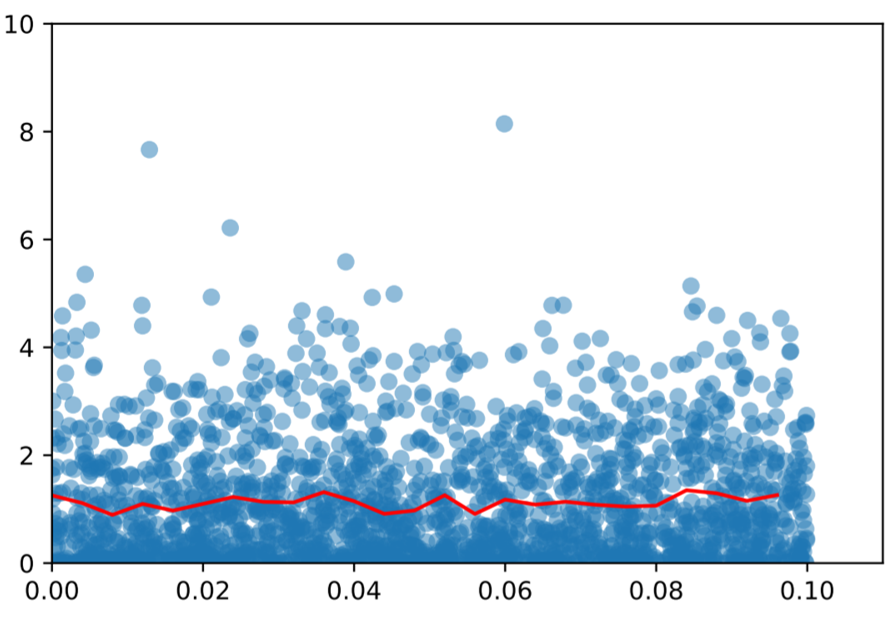Fig 4: $$\chi^2$$ values as a function of one 𝝐 parameter. The T2K model is not sensitive to these parameters in any interval of $$b_i$$ or $$\zeta_i$$. No further constraints are placed on them aside from those in Ref. .

Results from JUNO

Future experiments at the Jiangmen Underground Neutrino Observatory could place better constraints on this non-unitary model.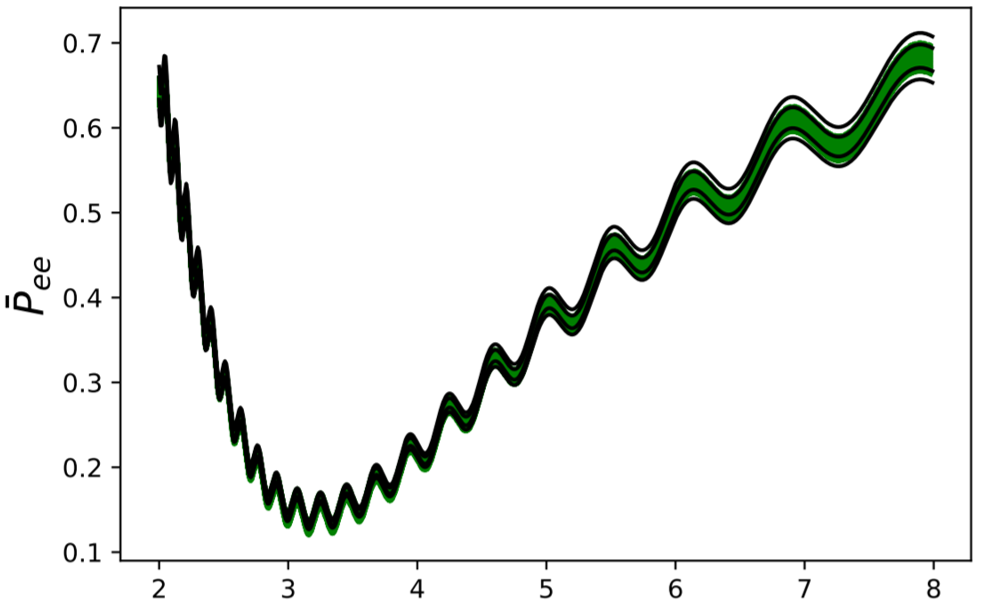Fig 5: Electron neutrino survival probability as a function of energy. The whole spectrum of energies is probed in JUNO, unlike in T2K. Black curves represent $$\pm 1\sigma$$ and $$\pm 2\sigma$$ uncertainties. Green areas indicated possible curves given varied non-unitary parameters.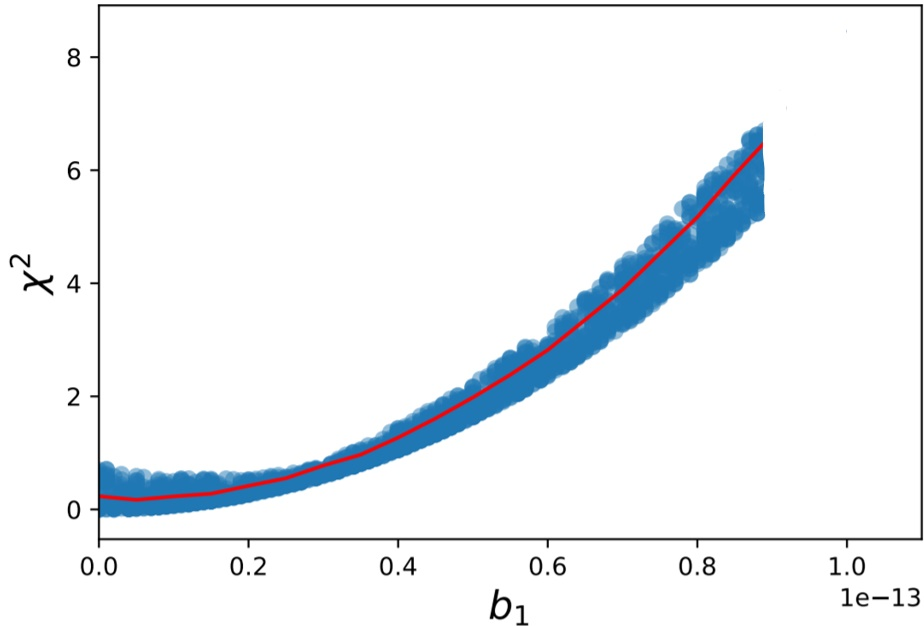Fig 6: $$\chi^2$$ values as a function of one $$b$$ parameter while all other parameters are turned off. This analysis constrains $$b_i \leq 8 \times 10^{-14}$$ eV. Previous studies [4,5] found such decay parameters to be constrained below $$10^{-13}$$ eV. This model is more sensitive than that of T2K.

References

 Hayato, Y. et al. 2003, Letter of Intent: Neutrino Oscillation Experiment at JHF, Tech. rep.

 An, F., et al. 2016, Jour. Phys. G. Nucl. Part. Phys., 43.

 Berryman, J. M., de Gouvêa, A., Hernández, D., & Oliveira, R. L. 2015, Phys. Let. B, 742, 74.

 Abrahão, T., Minakata, H., Nunokawa, H., & Quiroga, A. A. 2015, Jour. High Energy Phys., 2015, 1.

 Coloma, P., & Peres, L. 2017. arXiv:1705.03599[hep-ph]

Acknowledgments

This material is based upon work supported by the National Science Foundation under Grant No. AST-1757792, a Research Experiences for Undergraduates (REU) grant awarded to CIERA at Northwestern University. Any opinions, findings, and conclusions or recommendations expressed in this material are those of the author(s) and do not necessarily reflect the views of the National Science Foundation.

email: santos30 [at] purdue.edu